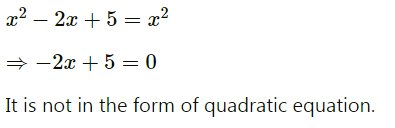is the following equation quadratic?x^{2} - 2x + 5 = x^{2} | FiloClass 10

Math

All topics595

Solution:
A quadratic equation in the form of595Connecting you to a tutor in 60 seconds.